# Motion problems + unit conversion - math problems

#### Number of problems found: 70

• Car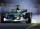Car is going 95 km per hour. How long distance goes in 1 minute?
• RPMAn electric motor makes 3,000 revolutions per minutes. How many degrees does it rotate in one second?
• Cyclist 12What is the average speed of a cycle traveling at 20 km in 60 minutes in km/h?
• Car loop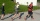The toy car runs at an average speed of 2 m/s. In a minute, it will pass the entire circuit five times. How long is the loop?
• The train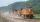The train passed the observer in the 20s and passed through a 1500m tunnel in 2 minutes. Calculate the speed (km/h) and train length.
• Passenger car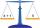Passenger car travels 1 km for 2.3 minutes, a truck during a 50 seconds long. How much is the speed of the truck less?
• Avg speed of flight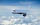The students vice adventure had a 2,367 km flight. If they travel time was 2 hours and 56 minutes, what was their average speed in kilometres per hour?
• Train and walker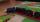Walker passes an hour 5 km. Express train per hour 100 km. Walker went 4 hours. How many minutes took the distance express train?
• Motion problemFrom Levíc to Košíc go car at speed 81 km/h. From Košíc to Levíc go another car at speed 69 km/h. How many minutes before the meeting will be cars 27 km away?
• Earth and Sun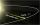Earth is 150 million km away from the Sun, 300,000 km of sunlight travels in 1 second. How long will the light come from the Sun to the Earth?
• Two busesThe first bus runs 15 minutes the second bus runs after 21 minutes. Together they both leave at 7:00 on Monday. When and what day will they meet?
• Two trainsThe train runs at speed v1 = 72 km/h. The passenger, sitting in the train, observed that a train long l = 75m in 3 s passed on the other track in the opposite direction. Calculate the speed of this train.
• Motorbike circuit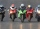On a 2,550 m long circuit, two motorcycles go at such speeds that they meet every minute if they go against each other and run every 5 minutes if they go in the same direction. Find their speeds.
• Two trains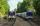Two trains go at each other. First at speed 40 km/h and the second at speed 60 km/h. At what distance will be 30 minutes before they will meet?The railroad runs parallel to the railway. The train travels at 36 km/h on track. In the opposite direction, the motorcycle rides at 90 km/h. The train passes in 3 seconds. How long is a train?
• Train speed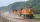The train speed is decreased during 50 sec from 72 km/h to 36 km/h. Assuming that the train movement is equally slowing, find the the acceleration and the distance that it travels at.
• Average speed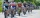The average speed of a pedestrian who walked 10 km was 5km/h, the average speed of a cyclist on the same track was 20km/h. In how many minutes did the route take more than a cyclist? Q
• Earth's diameter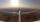The Earth's diameter on the equator is approximately 12750 km. How long does the Gripen flyover the Earth above the equator at 10 km if it is at an average speed of 1500 km / h?
• Average speedWhen the bus stops at bus stops driving average speed is 45 km/h. If it did not stop it drive at speed 54 km/h. How many minutes of every hour it spend at stops?
• Scale of mapJames travels one kilometer in 12 minutes. The route walked for half an hour measured on the map 5 cm. Calculate how many kilometers James walked for half an hour. Find the scale of the map.

Do you have an interesting mathematical word problem that you can't solve it? Submit a math problem, and we can try to solve it.

We will send a solution to your e-mail address. Solved examples are also published here. Please enter the e-mail correctly and check whether you don't have a full mailbox.

Please do not submit problems from current active competitions such as Mathematical Olympiad, correspondence seminars etc...

Motion problems - math word problems. Unit conversion - math word problems.